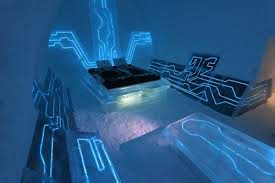Published on Sep 16, 2019

### Abstract

Electricity Theft Identification System project is microcontroller based Electricity Theft Identification system. This project will not only useful to electricity theft, and also it will be useful the monitor and transfer energy, calculating the fare, and using the prepaid card for paying the amount to an EB substation.

If suppose we implement the project for a complete network in an area, that will be consuming very huge amount and it can calculate the energy transmission and energy consumption. By using the above two values we can calculate the transmission loses we are going to implement an energy meter with wireless transmission for single house.

In a house to calculate the energy we have to monitor the existing energy meter reading. The energy meter readings are taken by an optical sensor, which is kept in between the slotted disk of the analog energy meter. Then the number of pulses will be counted.

Then the microcontroller will display the energy output in seven segment LED display. The accuracy of the meter depends on the existing meter.

The sim card is actually a memory card which is used to detect the amount from the card